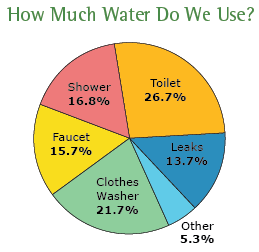# Pie Graph Worksheets

What Are Pie Graphs? The pie graph or pie chart is a special chart that uses slices to depict relative sizes of data. The chart is divided into different sectors, which determines the size of different values. The value of the entire pie is 100 percent. Now let's take an example. The following chart shows the water usage in our homes.Now, if we add the values of percentages, the value we will get is 100. This is probably the most used chart by organizations, given that it is easier to understand, and it shows us an exact percentage of data available in our sample space.

• ### Basic Lesson

Using a snap shot of a random pie graph students learn to field questions. The graph below shows the total hours per day worked by a group of employees for each month.

• ### Intermediate Lesson

Students begin to answer higher level questions based on the pie graph. Make a Pie graph for each set below. 1. Convert the data into percentages. 2. Multiply the percentage by 360 to get an angle. 3. Use compass to draw Circle. Draw radius. Place protractor on the circle so that 90 degrees are exactly above the center of circle. 4. Draw the section by using angles. Each time you add a section the radius to the line you just drew.

• ### Independent Practice 1

Students answer 20 questions using a random pie graphs.

• ### Independent Practice 2

The questions on this one are to see if they are paying attention to what they are reading.

• ### Homework Worksheet

Offers 8 questions for home and provides two examples.

• ### Skill Quiz

Students use a pie graphs to answer 10 quiz questions.

• ### Homework and Quiz Answer Key

Answers for the homework and quiz.